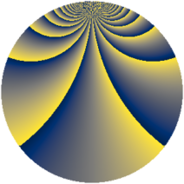# Properties

 Label 3549.2.crLevel $3549$ Weight $2$ Character orbit 3549.cr Rep. character $\chi_{3549}(211,\cdot)$ Character field $\Q(\zeta_{39})$ Dimension $4320$ Sturm bound $970$

# Related objects

## Defining parameters

 Level: $$N$$ $$=$$ $$3549 = 3 \cdot 7 \cdot 13^{2}$$ Weight: $$k$$ $$=$$ $$2$$ Character orbit: $$[\chi]$$ $$=$$ 3549.cr (of order $$39$$ and degree $$24$$) Character conductor: $$\operatorname{cond}(\chi)$$ $$=$$ $$169$$ Character field: $$\Q(\zeta_{39})$$ Sturm bound: $$970$$

## Dimensions

The following table gives the dimensions of various subspaces of $$M_{2}(3549, [\chi])$$.

Total New Old
Modular forms 11760 4320 7440
Cusp forms 11568 4320 7248
Eisenstein series 192 0 192

## Trace form

 $$4320q - 4q^{2} + 176q^{4} - 16q^{5} + 24q^{8} + 180q^{9} + O(q^{10})$$ $$4320q - 4q^{2} + 176q^{4} - 16q^{5} + 24q^{8} + 180q^{9} + 4q^{10} + 4q^{11} - 4q^{13} + 4q^{15} + 168q^{16} + 20q^{17} + 8q^{18} - 8q^{19} - 20q^{20} + 116q^{22} + 12q^{23} - 384q^{25} + 20q^{26} + 16q^{29} - 8q^{30} + 112q^{31} + 12q^{32} + 4q^{33} + 228q^{34} + 176q^{36} + 8q^{37} - 56q^{38} - 8q^{39} + 92q^{40} + 16q^{41} - 4q^{42} - 8q^{43} + 48q^{44} + 8q^{45} - 20q^{46} + 48q^{47} + 16q^{48} + 180q^{49} + 4q^{50} + 24q^{51} + 280q^{52} + 80q^{53} + 288q^{55} + 84q^{58} + 12q^{59} + 152q^{60} + 24q^{61} + 240q^{62} - 328q^{64} + 20q^{65} + 160q^{66} + 72q^{67} + 52q^{68} + 4q^{69} + 48q^{70} - 12q^{72} - 16q^{73} + 160q^{74} - 456q^{76} - 16q^{77} + 236q^{78} + 16q^{79} - 4q^{80} + 180q^{81} + 284q^{82} - 112q^{83} + 108q^{85} - 80q^{86} + 224q^{87} - 1004q^{88} - 48q^{89} - 8q^{90} + 8q^{91} - 120q^{92} + 144q^{94} - 184q^{95} - 40q^{96} - 4q^{97} - 4q^{98} - 8q^{99} + O(q^{100})$$

## Decomposition of $$S_{2}^{\mathrm{new}}(3549, [\chi])$$ into newform subspaces

The newforms in this space have not yet been added to the LMFDB.

## Decomposition of $$S_{2}^{\mathrm{old}}(3549, [\chi])$$ into lower level spaces

$$S_{2}^{\mathrm{old}}(3549, [\chi]) \cong$$ $$S_{2}^{\mathrm{new}}(169, [\chi])$$$$^{\oplus 4}$$$$\oplus$$$$S_{2}^{\mathrm{new}}(507, [\chi])$$$$^{\oplus 2}$$$$\oplus$$$$S_{2}^{\mathrm{new}}(1183, [\chi])$$$$^{\oplus 2}$$